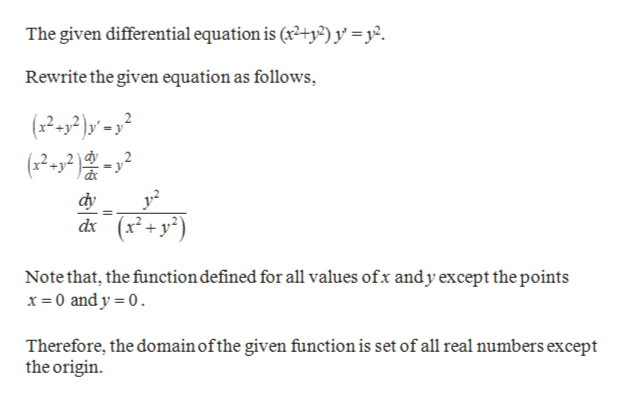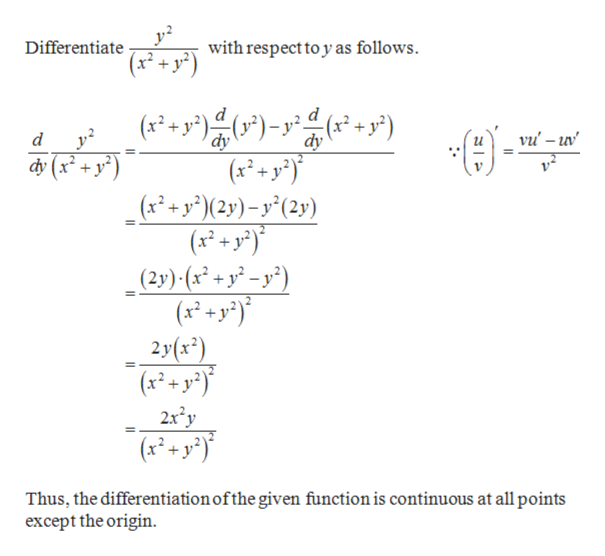# Determine a region of the ty-plane for which the given differential equation would have a unique solution whose graph passes through a point (x0, y0) in the region:(x2+y2)y'=y2

Question
94 views

Determine a region of the ty-plane for which the given differential equation would have a unique solution whose graph passes through a point (x0, y0) in the region:

(x2+y2)y'=y2

check_circle

Step 1help_outlineImage TranscriptioncloseThe given differential equation is (2+y2)y =. Rewrite the given equation as follows ر-)هر,.م dy (x+y) dx Note that, the function defined for all values ofx andy except the points x 0 and y 0 Therefore, the domain ofthe given function is set of all real numbers except the origin fullscreen
Step 2help_outlineImage TranscriptioncloseDifferen x+ y2) with respect to y as follows. iate d (y2 dy d d dy vu -uv (x+y) (xy(2y)-y°(2y) (x+ y _(2y) (x* + y? =y?) (x+y) 2y(x*) (xyy 2x2y 2+y Thus, the differentiation ofthe given function is continuous at all points except the origin fullscreen

### Want to see the full answer?

See Solution

#### Want to see this answer and more?

Solutions are written by subject experts who are available 24/7. Questions are typically answered within 1 hour.*

See Solution
*Response times may vary by subject and question.
Tagged in